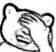# 博客

## UOJ开始记录测评历史

2021-02-19 19:41:16 By vfleaking

14年，vfk 在写 UOJ 的时候

• 大家还在争论 C++ 和 Pascal 孰优孰劣；g++ 最新版还是 4.8；C++ 11 还没有什么人写；
• 大家在为 NOI Linux 换成 Ubuntu 12.04 的内核而欢呼雀跃；Ubuntu 14.04 LTS 还是非常新潮的操作系统；
• Chrome 还没有满地跑；IE 还没有退出历史舞台；
• Bootstrap 4 还没有出；HTML 5 和 CSS 3 还有很多浏览器不支持；
• 王宏老师在 WC 上关于IOI近年的非传统题演讲还言犹在耳；OI 界掀起了一波出非传统题的风潮；提交答案题我记得还只有 Contest Hunter 一家能测……

vfk 当时以为，我们 OIer 只要固定一组系统配置，那么对大家的程序就都是公平的。。并且 vfk 当时不知为何还产生了 “计算机的世界过去迭代了这么多轮了，所以未来变化不大了” 的错觉。。所以当时就固定下来了在当时看来还很新潮但现在看来已经有点老旧的配置。。。## 评论

EntropyIncreaser

YuHaoXiang
vfk 什么时候能在 github 上开源呢[期待]
Encounter_you

fhqTreap
qpzc
LDNNB
@mike
LDNNB
@vfleaking
zqs
qp orz
ChthollyODT
$x^x^x^x^x^x^x^x^x^x^x^x^x^x^x^x^x^x^x^x^x^x^x^x^x^x^x^x^x^x^x^x^x^x^x^x^x^x^x^x^x^x^x^x^x^x^x^x^x^x^x^x^x^x^x^x^x^x^x^x^x^x^x$